UIHistories Project: A History of the University of Illinois by Kalev Leetaru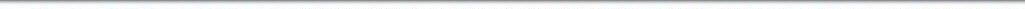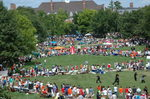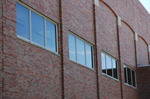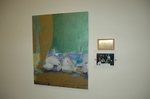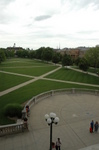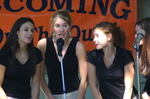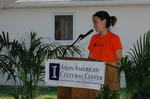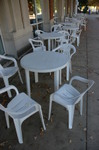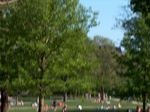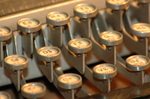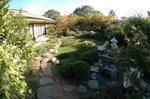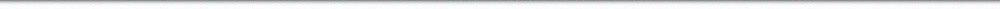N A V I G A T I O N D I G I T A L L I B R A R Y

## Repository: UIHistories Project: Board of Trustees Minutes - 1896 [PAGE 216]

Caption: Board of Trustees Minutes - 1896
This is a reduced-resolution page image for fast online browsing.

< Previous Page [Displaying Page 216 of 371] Next Page >
[VIEW ALL PAGE THUMBNAILS]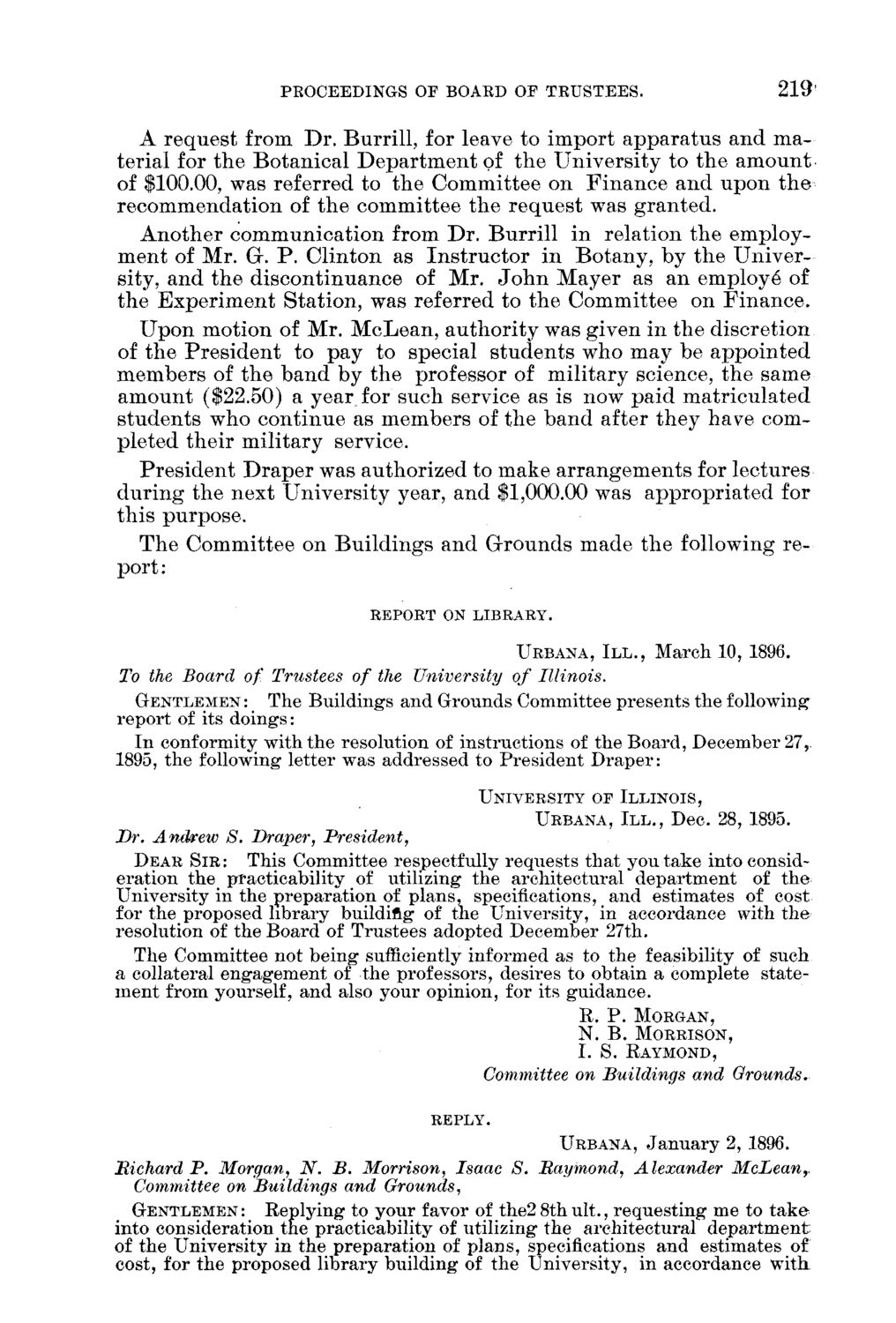### EXTRACTED TEXT FROM PAGE:

PROCEEDINGS OF BOAED OF TRUSTEES.

219

A r e q u e s t from D r . B u r r i l l , for leave to i m p o r t a p p a r a t u s a n d m a terial for t h e B o t a n i c a l D e p a r t m e n t of t h e U n i v e r s i t y to t h e a m o u n t of \$100.00, was referred to t h e C o m m i t t e e on F i n a n c e a n d u p o n t h e r e c o m m e n d a t i o n of t h e c o m m i t t e e t h e r e q u e s t was g r a n t e d . A n o t h e r c o m m u n i c a t i o n from D r . B u r r i l l in relation t h e e m p l o y m e n t of M r . Gr. P . C l i n t o n as I n s t r u c t o r i n B o t a n y , b y t h e U n i v e r sity, a n d t h e d i s c o n t i n u a n c e of M r . J o h n M a y e r as a n employe* of t h e E x p e r i m e n t S t a t i o n , was referred to t h e C o m m i t t e e on F i n a n c e , U p o n m o t i o n of M r . M c L e a n , a u t h o r i t y was given in t h e d i s c r e t i o n of t h e P r e s i d e n t to p a y t o special s t u d e n t s w h o m a y be a p p o i n t e d m e m b e r s of t h e b a n d b y t h e professor of m i l i t a r y science, t h e s a m e a m o u n t (\$22.50) a year for s u c h service as is now p a i d m a t r i c u l a t e d s t u d e n t s w h o c o n t i n u e as m e m b e r s of t h e b a n d after t h e y have completed t h e i r m i l i t a r y service. P r e s i d e n t D r a p e r was a u t h o r i z e d to m a k e a r r a n g e m e n t s for lectures d u r i n g t h e n e x t U n i v e r s i t y year, a n d \$1,000.00 was a p p r o p r i a t e d for t h i s purpose. T h e C o m m i t t e e on B u i l d i n g s a n d G r o u n d s m a d e t h e following r e port :

REPORT ON LIBRARY.

URBANA, I I I . , March 10, 1896.

To the Board of Trustees of the University of Illinois. GENTLEMEN: The Buildings and Grounds Committee presents the following report of its doings: In conformity with the resolution of instructions of the Board, December 27,. 1895, the following letter was addressed to President Draper:

UNIVERSITY OF ILLINOIS, URBANA, I I I . , Dec. 28, 1895.

Dr. Andrew S. Draper, President, DEAR S I R : This Committee respectfully requests that you take into consideration the practicability of utilizing the architectural department of the University in the preparation of plans, specifications, and estimates of cost for the proposed library building of the University, in accordance with the resolution of the Board of Trustees adopted December 27th. The Committee not being sufficiently informed as to the feasibility of such a collateral engagement of the professors, desires to obtain a complete statement from yourself, and also your opinion, for its guidance.

R. P . MORGAN, N. B. MORRISON, I. S. EAYMOND,

Committee on Buildings and Grounds,